Pay

## Car Renting Process UML Diagram

4
0
1
publish time: 2020-12-28Chritsmas Card

This is an example of car renting process UML composite structure diagram. This type of UML diagram is not commonly used because its function is very specific. It only represents the internal structure of a class and the relations between different class components.

See More Related TemplatesThis is an example of a UML diagram about adding events to the calendar.
UML Diagram - Add event to calendar
23
1
0easy diagrams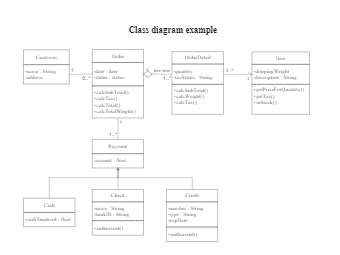This is an example of a uml class diagram.
Class diagram example
178
5
4easy diagrams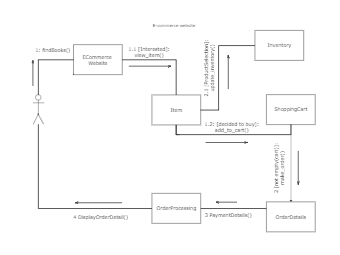This is an example of a UML collaboration diagram.
ecommerce website - UML diagram
14
1
0easy diagrams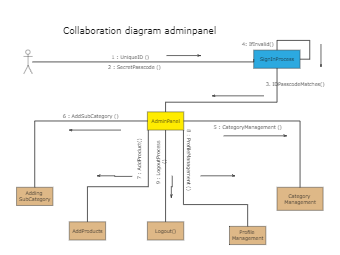This is an example of a UML collaboration diagram about adminpanel.
Collaboration diagram adminpanel
7
1
0easy diagrams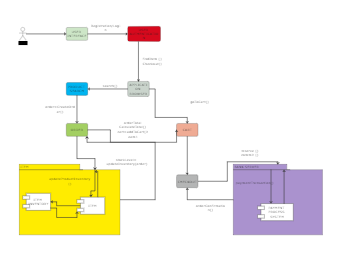This is an example of a UML communication diagram.
Communication diagram example
8
1
0easy diagrams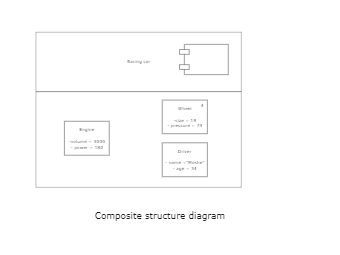This is an example of a composite structure diagram.
Composite structure diagram
3
0
0easy diagrams